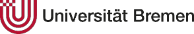# Teaching

### Scheduling Theory

#### Course in Winter 2017/18 6 ECTS

Scheduling involves the timely allocation of tasks to scarce resources with the objective to minimize some cost function. Such problems appear in various applications, e.g., in production planning, logistics, project management, or when operating computing systems. This lecture will cover: a classification of scheduling models, complexity of scheduling problems, the design and analysis of exact and approximation algorithms, single and parallel machine models, flow shop and open shop problems. The focus lies on the design and mathematical analysis of algorithms using combinatorial techniques, dynamic programming and linear programming.

 Lecturer: Assistent: Prof. Dr. Nicole Megow (Email) M.Sc. Franziska Eberle (Email) Lecture: Mon 14-16 in room MZH 1110 (Megow) Exercise: Mon 16-18 in room MZH 1110 (Eberle) The first lecture is on Monday, October 23, 2017. The first exercise class is on Monday, October 30, 2017 and starts at 16:00 (sharp).
Date Topic Material
23/10/2017 Introduction, three field notation, single machine, Smith's rule orga slides, introduction, notes by J. Winter
30/10/2017 Single machine: 1|(prec)|L_max (EDF), 1|prec|f_max (Lawler's algorithm) notes by T. Hahn
06/11/2017 Single machine: 1||sum U_j (Moore Hodgson), parallel machines: P||sum C_j (SPT), R||sum C_j (matching), P|pmtn|C_max (McNaughton Wrap around) notes by J. Cepok
13/11/2017 no lecture, no exercise
20/11/2017 Q|pmtn|C_max (Horvath, Lam, Sethi 1977), short intro to linear programming notes by M. Speer
27/11/2017 Complexity of scheduling problems notes by T. Hahn
04/12/2017 Intro approximation algorithms, P|(prec)|C_max: list scheduling, LPT
11/12/2017 Optimal poly-time alg for P2|prec,p_j=1|C_max, fast single machine relaxation for P|r_j,pmtn|sum w_jC_j notes by T. Haslop
18/12/2017 P|r_j,pmtn|sum w_jC_j preemptive WSPT 2-approx, conversion technique, alpha-points
08/01/2018 randomized algorithms, 1|r_j|sum w_jC_j randomized scheduling by alpha-points [Chekuri et al.], [Skutella]
15/01/2018 Bin packing, PTAS for makespan minimization notes, paper by Hochbaum, Shmoys
22/01/2018 FPTAS for makespan minimization, FPTAS for knapsack notes
29/01/2018 LP based algorithms (P||sum w_jC_j) notes
Literature: The course is based on parts of the literature below and recent research articles that will be added later.
• M. L. Pinedo. Scheduling: Theory, Algorithms, and Systems. Springer, 2012.
• J. Y.-T. Leung. Handbook of Scheduling: Algorithms, Models, and Performance Analysis. Chapman & Hall/CRC, 2004.
• F. Jaehn and E. Pesch: Ablaufplanung. Einführung in Scheduling. Springer, 2014.